Next: Charge Conjugation Up: Interaction with the Electromagnetic Previous: Gauge Invariance

## Electromagnetic Coupling

The coupling of scalar particles to an electromagnetic field is done using the gauge invariant approach explained in the previous section. Using the minimal coupling,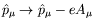, and writing the Klein-Gordon equation so that all terms involving the electromagnetic potential appear on one side, we have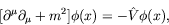(4.69)

where the potential operator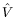is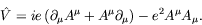(4.70)

As the Klein-Gordon equation is second order, the coupling term has a quite complicated structure. It contains gradients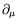and moreover is nonlinear in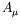because of the quadratic last term.

Using minimal coupling, the conserved current becomes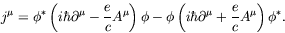(4.71)

Multiplying by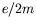to get the usual normalization gives the time and space components of the conserved current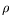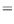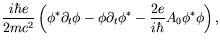(4.72)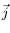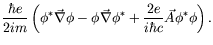(4.73)

Returning to our initial normalization and using natural units, we have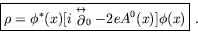(4.74)

For a stationary state,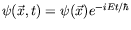, equation 4.72 gives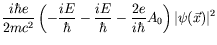(4.75)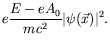(4.76)

If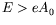,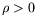and the charge density has the same sign as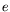of the particle. But if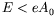,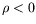and the charge density has the opposite sign asof the particle. In this case the field is strong and we would need to invoke field theory to show that particles are created in this case.Next: Charge Conjugation Up: Interaction with the Electromagnetic Previous: Gauge Invariance
Douglas M. Gingrich (gingrich@ ualberta.ca)
2004-03-18